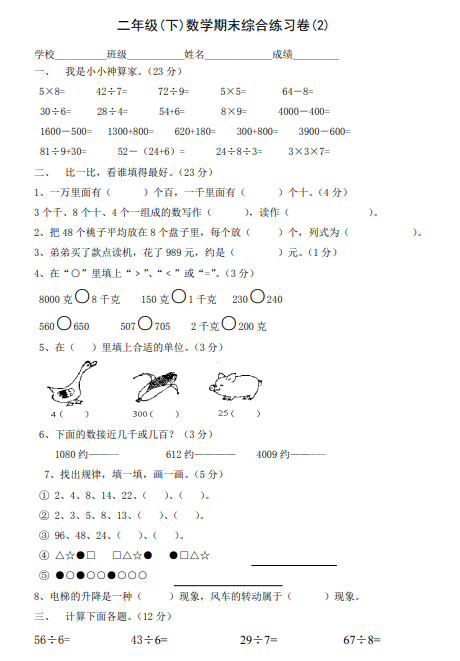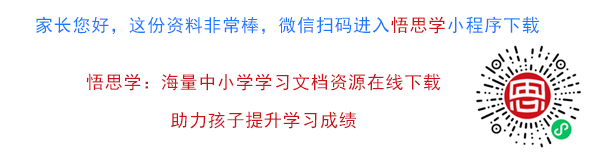5×8= 42÷7= 72÷9= 5×5= 64－8=
30÷6= 28÷4= 54+6= 8×9= 4000－400=
1600－500= 1300+800= 620+180= 300+800= 3900－600=
81÷9+30= 52－（24+6）= 24÷8÷3= 3×3×7=

1、一万里面有（ ）个百，一千里面有（ ）个十。（4 分）##### 评论信息